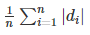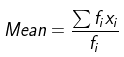• +91 9971497814
• info@interviewmaterial.com

# RD Chapter 32- Statistics Ex-32.3 Interview Questions Answers

### Related Subjects

Question 1 :

Compute the meandeviation from the median of the following distribution:

 Class 0-10 10-20 20-30 30-40 40-50 Frequency 5 10 20 5 10

To find the mean deviation from the median, firstly let uscalculate the median.

Median is the middle term of the Xi,

Here, the middle term is 25

So, Median = 25

 Class Interval xi fi Cumulative Frequency |di| = |xi – M| fi |di| 0-10 5 5 5 20 100 10-20 15 10 15 10 100 20-30 25 20 35 0 0 30-40 35 5 91 10 50 40-50 45 10 101 20 200 Total = 50 Total = 450

MD== 1/50 × 450

= 9

Themean deviation is 9

Question 2 :

Find the meandeviation from the mean for the following data:

(i)

 Classes 0-100 100-200 200-300 300-400 400-500 500-600 600-700 700-800 Frequencies 4 8 9 10 7 5 4 3

(ii)

 Classes 95-105 105-115 115-125 125-135 135-145 145-155 Frequencies 9 13 16 26 30 12

(i)

To find the mean deviation from the mean, firstly let uscalculate the mean.

By using the formula,= 17900/50

= 358

 Class Interval xi fi Cumulative Frequency |di| = |xi – M| fi |di| 0-100 50 4 200 308 1232 100-200 150 8 1200 208 1664 200-300 250 9 2250 108 972 300-400 350 10 3500 8 80 400-500 450 7 3150 92 644 500-600 550 5 2750 192 960 600-700 650 4 2600 292 1168 700-800 750 3 2250 392 1176 Total = 50 Total = 17900 Total = 7896

N = 50

MD=n= 1/50 × 7896

= 157.92

Themean deviation is 157.92

(ii)

To find the mean deviation from the mean, firstly let uscalculate the mean.

By using the formula,= 13630/106

= 128.58

 Class Interval xi fi Cumulative Frequency |di| = |xi – M| fi |di| 95-105 100 9 900 28.58 257.22 105-115 110 13 1430 18.58 241.54 115-125 120 16 1920 8.58 137.28 125-135 130 26 3380 1.42 36.92 135-145 140 30 4200 11.42 342.6 145-155 150 12 1800 21.42 257.04 N = 106 Total = 13630 Total = 1272.6

N = 106

MD== 1/106 × 1272.6

= 12.005

Themean deviation is 12.005

Question 3 :

Compute meandeviation from mean of the following distribution:

 Marks 10-20 20-30 30-40 40-50 50-60 60-70 70-80 80-90 No. of students 8 10 15 25 20 18 9 5

To find the mean deviation from the mean, firstly let uscalculate the mean.

By using the formula,= 5390/110

= 49

 Class Interval xi fi Cumulative Frequency |di| = |xi – M| fi |di| 10-20 15 8 120 34 272 20-30 25 10 250 24 240 30-40 35 15 525 14 210 40-50 45 25 1125 4 100 50-60 55 20 1100 6 120 60-70 65 18 1170 16 288 70-80 75 9 675 26 234 80-90 85 5 425 36 180 N = 110 Total = 5390 Total = 1644

N = 110

MD== 1/110 × 1644

= 14.94

Themean deviation is 14.94

Question 4 :

The agedistribution of 100 life-insurance policy holders is as follows:

 Age (on nearest birthday) 17-19.5 20-25.5 26-35.5 36-40.5 41-50.5 51-55.5 56-60.5 61-70.5 No. of persons 5 16 12 26 14 12 6 5

Calculate the meandeviation from the median age.

To find the mean deviation from the median, firstly let uscalculate the median.

N = 96

So, N/2 = 96/2 = 48

The cumulative frequency just greater than 48 is 59, and thecorresponding value of x is 38.25

So, Median = 38.25

 Class Interval xi fi Cumulative Frequency |di| = |xi – M| fi |di| 17-19.5 18.25 5 5 20 100 20-25.5 22.75 16 21 15.5 248 36-35.5 30.75 12 33 7.5 90 36-40.5 38.25 26 59 0 0 41-50.5 45.75 14 73 7.5 105 51-55.5 53.25 12 85 15 180 56-60.5 58.25 6 91 20 120 61-70.5 65.75 5 96 27.5 137.5 Total = 96 Total = 980.5

N = 96

MD== 1/96 × 980.5

= 10.21

Themean deviation is 10.21

Question 5 :

Find the meandeviation from the mean and from a median of the following distribution:

 Marks 0-10 10-20 20-30 30-40 40-50 No. of students 5 8 15 16 6

To find the mean deviation from the median, firstly let uscalculate the median.

N = 50

So, N/2 = 50/2 = 25

The cumulative frequency just greater than 25 is 58, and thecorresponding value of x is 28

So, Median = 28

By using the formula to calculate Mean,= 1350/50

= 27

 Class Interval xi fi Cumulative Frequency |di| = |xi – Median| fi |di| FiXi |Xi – Mean| Fi |Xi – Mean| 0-10 5 5 5 23 115 25 22 110 10-20 15 8 13 13 104 120 12 96 20-30 25 15 28 3 45 375 2 30 30-40 35 16 44 7 112 560 8 128 40-50 45 6 50 17 102 270 18 108 N = 50 Total = 478 Total = 1350 Total = 472

Mean deviation from Median = 478/50 = 9.56

And, Mean deviation from Median = 472/50 = 9.44

TheMean Deviation from the median is 9.56 and from mean is 9.44.

Todays Deals### RD Chapter 32- Statistics Ex-32.3 Contributorskrishan

Name:
Email:

# Latest News# 9000 interview questions in different categories